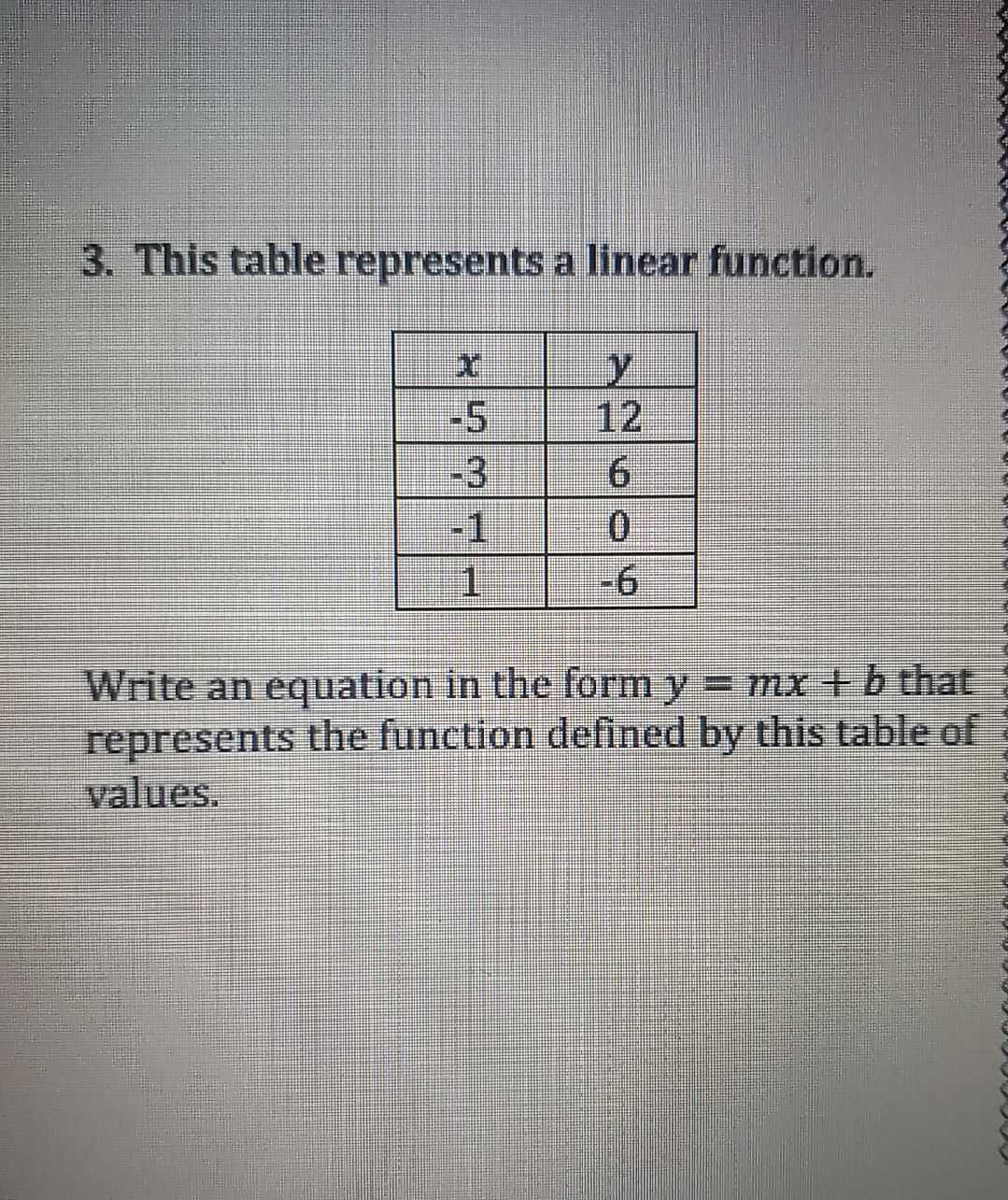Still have math questions?

Algebra
Questionwrite an equation in the form y=mx+b that represents the function defined by this table of values

(-5,12),(-3,6),(-1,0),(1,-6)